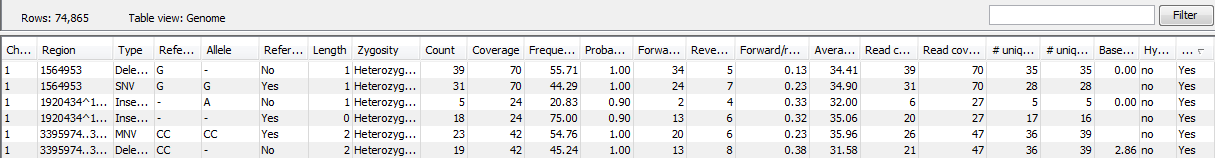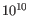## The variant track output

The variant track contains information on each of the variants called, including reference alleles. When opened in the table view there is a number of columns for each of the variants (see figure 26.17).Figure 26.17: A variant track shown in the table view.

The contents of these are explained in Variant tracks, except for the following:

Probability
The contents of the Probability column (for Low Frequency and Fixed Ploidy Variant Detection tool only) depend on the variant detection tool that produced and the type of variant:
• In the Fixed Ploidy Variant Detection Tool, the probability in the resulting variant track's 'Probability' column is NOT the probability referred to in the wizard. The probability referred to in the wizard is the required minimum (posterior) probability that the site is NOT homozygous for the reference. The probability in the variant track 'Probability' column is the posterior probability of the particular site-type called. The fixed ploidy tool calculates the probability of the different possible configurations at each site. So using this tool, for single site variants the probability column just contains this quantity (for variants that span multiple positions see below).
• The Low Frequency Variant Detection tool makes statistical tests for the various possible explanations for each site. This means that the probability for the called variant must be estimated separately since it is not part of the actual variant calling. This is done by assigning prior probabilities to the various explanations for a site in a way that makes the probability for two explanations equal in exactly the situation where the statistical test shifts from preferring one explanation to the other. For a given single site variant, the probability is then calculated as the sum of probabilities for all the explanations containing that variant. So if a G variant is called, the reported probability is the sum of probabilities for these configurations: G, A/G, C/G, G/T, A/C/G, A/G/T, C/G/T, and A/C/G/T (and also all the configurations containing deletions together with G).
For multi position variants, an estimate is made of the probability of observing the same read data if the variant did not exist and all observations of the variant were due to sequencing errors. This is possible since a sequencing error model is found for both the fixed ploidy and rare variant tools. The probability column contains one minus this estimated probability. If this value is less than 50%, the variant might as well just be the result of sequencing errors and it is not reported at all.

Read count
The number of 'countable' reads supporting the allele. Only 'countable' reads are considered (see under 'Count' above for an explanation of 'countable' reads). Note that each read in an overlapping pair contribute 1. To view the reads in pairs in a reads track as single reads, check the 'Disconnect paired reads' option in the side-panel of the reads track. (Please see the column 'Count' above for a column that reports the value for 'fragments' rather than for 'reads').
Read coverage
The read coverage at this position. Only 'countable' reads are considered (see under 'Count' above for an explanation of 'countable' reads). Note that each read in an overlapping pair contribute 1. To view the reads in pairs in a reads track as single reads, check the 'Disconnect paired reads' option in the side-panel of the reads track. (Please see the column 'Coverage' above for a column that reports the value for 'fragments' rather than for 'reads').
# Unique start positions
The number of unique start positions for 'countable' fragments that support the variant. This value can be important to look at in cases with low coverage. If all reads supporting the variant have the same start position, you could suspect that it is a result of an amplification error.
# Unique end positions
The number of unique end positions for 'countable' fragments that support the variant. This value can be important to look at in cases with low coverage. If all reads supporting the variant have the same end position, you could suspect that it is a result of an amplification error.
BaseQRankSum
The BaseQRankSum column contains an evaluation of the quality scores in the reads that have a called variant compared with the quality scores of the reference allele. Reference alleles and variants for which no corresponding reference allele is called do not have a BaseQRankSum value. The score is a z-score derived using the Mann-Whitney U test, so a value of -2.0 indicates that the observed qualities for the variant are two standard deviations below what would be expected if they were drawn from the same distribution as the reference allele qualities. A negative BaseQRankSum indicates a variant with lower quality than the reference variant, and a positive z-score indicates higher quality than the reference.
Read position test probability
The test probability for the test of whether the distribution of the read positions variant in the variant carrying reads is different from that of all the reads covering the variant position.
Read direction test probability
Tests whether the distribution among forward and reverse reads of the variant carrying reads is different from that of all the reads covering the variant position. This value reflects a balanced presence of the variant in forward and reverse reads (1: well-balanced, 0: un-balanced). This p-value is based on a statistic that we assume follows a Chi-square(df=2) distribution under the null hypothesis of the variant having equal frequency on reads from both direction. Note that GATK uses a Fisher's exact test for the same purpose. The difference between both approaches lead to a potential overestimation of p-values output by the workbench's variant detection tools.
Hyper-allelic
Basic and Fixed Ploidy Variant detectors only: Contains "yes", if the site contains more variants than the user-specified ploidy predicts, "no" if not.
Genotype
Fixed Ploidy only: Contains the most probable genotype for the site.
Homopolymer
The column contains "Yes" if the variant is likely to be a homopolymer error and "No" if not. This is assessed by inspecting all variants in homopolymeric regions longer than 2. A variant will get the mark "yes" if it is a homopolymeric length variation of the reference allele, or a length variation of another variant that is a homopolymeric variation of the reference allele. When several overlapping homopolymeric variants are identified, all except the most frequent are marked as being homopolymer. However, if one of the overlapping, homopolymeric variants is the reference allele, then all of them are marked as homopolymer.
QUAL
Measure of the significance of a variant, i.e., a quantification of the evidence (read count) supporting the variant, relative to the coverage and what could be expected to be seen by chance, given the error rates in the data. The mathematical derivation of the value is depends on the set of probabilities of generating the nucleotide pattern observed at the variant site (1) by sequencing errors alone and (2) under the different allele models of the variant caller allows. Qual is calculated as -10log10(1-p), p being the probability that a particular variant exists in the sample. Qual is capped at 200 for p=1, with 200: highly significant, 0: insignificant. In rare cases, the Qual value cannot be calculated for specific variant and as a result the Qual field will be empty. This value is necessary for certain downstream analyses of the data after export in vcf format. A QUAL value of 10 indicates a 1 in 10 chance that the called variant is an error, while a QUAL of 100 indicates a 1 inchance that the called variant is an error.